# Gay-Lussac's Law

Back to Gas Laws

### Gay-Lussac's Law

Gay-Lussac's Pressure-Temperature Law is more often called Amontons' Law of Pressure-Temperature, because it was previously discovered by Guillaume Amontons between 1700 and 1702. The law states that pressure of a gas is directly related (proportional) to it's temperature. In other words, as the temperature goes up, the pressure also goes up. Increased temperature is the result of adding heat. This increases the kinetic energy of the gas particles, so they are moving faster. They strike each other and the walls of their container more often and with more force, so the pressure goes up.

Gay-Lussac's Law can be expressed in three ways: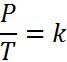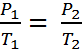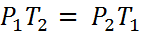Equation #1 Equation #2 Equation #3
The first equation leads to the second and third.   Here is how.  Given that
P is the pressure of the gas
T is the absolute temperature of the gas (measured in kelvin).
k is a constant (which depends on the units of P & T)

If the ratio (or fraction) of P and T is always a constant, then we can use an initial pressure and temperature, P1 and T1 to solve for pressure or temperature at another set of conditions, P2 or T2. This is because if P1/T1 = k, it must also be true that,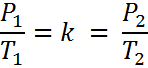We can remove “k” and we get Equation #2.  A little rearranging give us Equation #3.

It is important to note that you cannot solve this equation with temperatures in °C. You must convert to absolute temperature, or Kelvin (K). This is easy enough to do because, K = °C + 273. Also, pressures can be measured in any units, but whatever they are, both pressures must be in the same units.

Most students find the form of Equation #2 easiest to use. Consider the following word problem:

A gas with a pressure of 2.5 atmospheres and a temperature of 350 Kelvin is cooled to a temperature of 280 Kelvin. What is the new pressure?

First we identify the variables, P1=2.5 atm, T1=350K, T2 = 280 K and P2 is unknown. The temperatures absolutely must be in Kelvin. If the temperatures are in °C, simply add 273° to get the temperature in Kelvin. Now, we substitute into Equation #2 and get: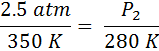From here, we just cross-multiply the two circled numbers and then divide by the third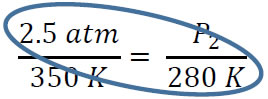P2 = (280K x 2.5 atm) / 350K = 2.0 atm. You can subsitute and cross multiply to solve for any of the four variables.

#### Practice

Click on the image above to go go to a site where you can practice Gay-Lussac's Law probems in an infinite number of combinations

Joseph Luis Gay-Lussac was a french chemist and physicist well known for two laws that bear his name. The first, his Pressure-Temperature Law, was actually discovered earlier by Guillaume Amonron, who was working on an air thermometer. It is now more commonly called Amontons' Pressure-Temperature Law.

The second law that bears his name is Gay-Lussac's law of combining volumes, which states, "The ratio between the volumes of the reactant gases and the products can be expressed in simple whole numbers."

He found that two volumes of hydrogen and one volume of oxygen would combine to form two volumes of water vapor. This simple, whole number ratio also matches the molar ratios found in the balanced chemical equation, though volume ratios apply only to gases. His results were published in 1808.

His experimental results led Amadeo Avogadro (yes, that Avogadro) to theorize that at the same temperature and pressure, equal volumes of gas contain an equal number of molecules. This led eventually to Avogadro's number, though it was not until the 1860's that Avogadro's ideas were accepted.

Gay-Lussac made other contributions to science, including an improved burrette, the recognition of iodine as an element, and new methods of quantitative measurement.#### Worksheets

Here are some worksheets with problems about Gay-Lussac's Law.#### PowerPoints

Here you can find some PowrPoints covering Gay-Lussac's Law.#### External Videos

There are a few sources of information about Gas Laws on the internet. Here are a few of them